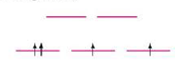# Which of the following crystal field diagram(s) is(are) correct for the complex given? a. Zn(NH 3 ) 4 2+ (tetrahedral) b. Mn(CN) 6 3− (strong field) c. Ni(SN) 4 2− (square planar, diamagnetic)### Chemistry: An Atoms First Approach

2nd Edition
Steven S. Zumdahl + 1 other
Publisher: Cengage Learning
ISBN: 9781305079243

#### Solutions

Chapter
Section### Chemistry: An Atoms First Approach

2nd Edition
Steven S. Zumdahl + 1 other
Publisher: Cengage Learning
ISBN: 9781305079243
Chapter 20, Problem 87CWP
Textbook Problem
6 views

## Which of the following crystal field diagram(s) is(are) correct for the complex given?a. Zn(NH3)42+ (tetrahedral)b. Mn(CN)63− (strong field)c. Ni(SN)42− (square planar, diamagnetic)(a)

Interpretation Introduction

Interpretation: The correct crystal field diagram for the given complex is to be identified.

Concept introduction: The electrons in the d orbital of a transition metal split into high and low energy orbitals when ligands are attached to it. The energy difference between these two levels depends upon the properties of both metal and the ligands. If the ligand is strong, then splitting will be high and the complex will be low spin. If the ligand is weak, then splitting will be less and the complex will be high spin.

To determine: If the given crystal field diagram for Zn(NH3)42+ is correct or not.

The given crystal field diagram for Zn(NH3)42+ is not correct.

### Explanation of Solution

Explanation

The given crystal field diagram for Zn(NH3)42+ (tetrahedral) is,

Figure 1

Although not pointing exactly at the ligands, the dxy , dyz and dxz orbitals are closer to the point charges than are the dz2 and dx2y2 orbitals in tetrahedral complexes

(b)

Interpretation Introduction

Interpretation: The correct crystal field diagram for the given complex is to be identified.

Concept introduction: The electrons in the d orbital of a transition metal split into high and low energy orbitals when ligands are attached to it. The energy difference between these two levels depends upon the properties of both metal and the ligands. If the ligand is strong, then splitting will be high and the complex will be low spin. If the ligand is weak, then splitting will be less and the complex will be high spin.

To determine: If the given crystal field diagram for Mn(CN)63+ is correct or not.

(c)

Interpretation Introduction

Interpretation: The correct crystal field diagram for the given complex is to be identified.

Concept introduction: The electrons in the d orbital of a transition metal split into high and low energy orbitals when ligands are attached to it. The energy difference between these two levels depends upon the properties of both metal and the ligands. If the ligand is strong, then splitting will be high and the complex will be low spin. If the ligand is weak, then splitting will be less and the complex will be high spin.

To determine: If the given crystal field diagram for Ni(CN)42- is correct or not.

### Still sussing out bartleby?

Check out a sample textbook solution.

See a sample solution

#### The Solution to Your Study Problems

Bartleby provides explanations to thousands of textbook problems written by our experts, many with advanced degrees!

Get Started

Find more solutions based on key concepts
All of the following are potential benefits of regular physical activity except improved body composition. lowe...

Nutrition: Concepts and Controversies - Standalone book (MindTap Course List)

What are the products of the acid hydrolysis of the local anesthetic lidocaine?

Chemistry for Today: General, Organic, and Biochemistry

1. What characteristics distinguish animals from plants and fungi?

Biology: The Dynamic Science (MindTap Course List)

Why are titanium oxide features visible in the spectra of only the coolest stars?

Horizons: Exploring the Universe (MindTap Course List)

What is the pH of a solution with a hydrogen ion concentration of 3105M?

Introductory Chemistry: An Active Learning Approach

You are standing on a saucer-shaped sled at rest in the middle of a frictionless ice rink. Your lab partner thr...

Physics for Scientists and Engineers, Technology Update (No access codes included)